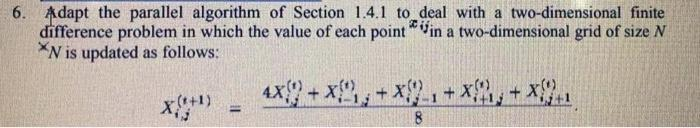# (Solved): 6. Adapt the parallel algorithm of Section $$1.4 .1$$ to deal with a two-dimensional finite diffe ...6. Adapt the parallel algorithm of Section $$1.4 .1$$ to deal with a two-dimensional finite difference problem in which the value of each point $${ }^{x_{i j}}$$ in a two-dimensional grid of size $$N$$ $X_{i, j}^{(t+1)}=\frac{4 X_{i, j}^{(t)}+X_{i-1, j}^{(t)}+X_{i, j-1}^{(t)}+X_{i+1, j}^{(t)}+X_{i, j+1}^{(t)}}{8}$

We have an Answer from Expert